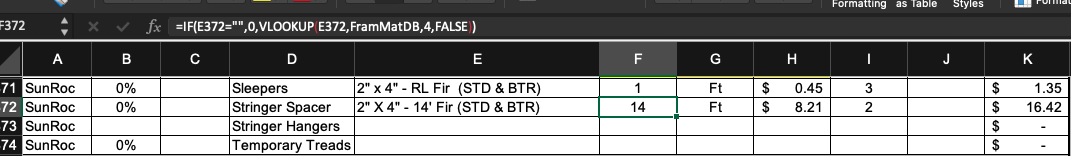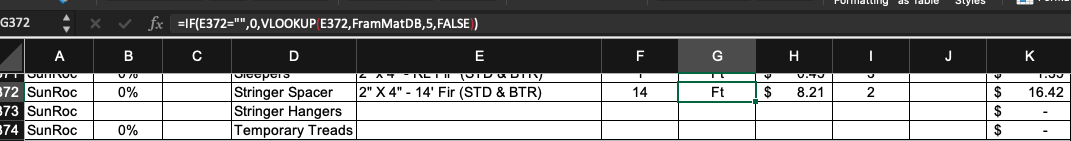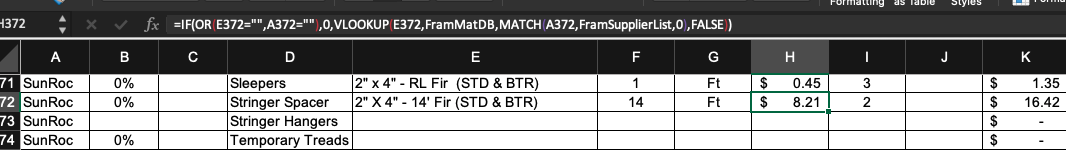# How to Complete Materials Estimates

## Material Size, Unit, and Unit Cost Definitions

When estimating materials in the Estimating Workbook, Size, Units, Unit Cost, Quantity, and Waste must all be calculated and accounted for. It is vital to understand what each of these terms mean in the context of construction estimating.

 Size The amount of material included in a single unit purchased from a supplier. Unit The way material is measured and sold by a supplier. Examples include linear feet, cubic yards, complete units, square feet, etc. Unit Cost How much a single unit of the material costs to purchase from a supplier. Quantity The number of items required to complete a specific construction task based on the quantity takeoff from the building plans. Waste The percentage of material that is anticipated to be unused or unusable due to error or unforeseen circumstances.

### Soft Drink Example

You plan to host a party to watch a sporting event on television with friends. Seven people will be present, including yourself.

You will provide beverages to your guests, who all prefer the same soft drink. You anticipate that over the course of the event, each person will drink three soft drinks. You know from experience that some of your guests will not finish their drinks, and that some may drink more than three.

You go to the store, and find that the soft drink is only sold in packages of six cans for $3.50 per package. You decide to purchase four packages of soft drinks. If you were to use the construction estimating definitions of the terms above, they would fit this example as follows: • Material - Preferred Soft Drink • Size - 6 • Units - cans/pk • Unit Cost -$3.50
• Quantity - 4

The Size is listed as 6 because the Unit is defined as cans/pk. If the Unit were defined as pack, the Size would be 1. This is problematic because you might not know how many cans are included in a pack, which is why the Unit is defined as cans/pk.

You intend for each of the seven attendees to drink three soft drinks. If you purchase four packages of six cans, you will have 24 total cans for the event. This is the minimum you must purchase to provide three cans to each attendee, and there should be three cans left over. This is why the Quantity is listed as 4.

### Shoe Example

You need a new pair of shoes. You go to the store and find a pair that you like. It costs $65.00. If you were to use the construction estimating definitions of the terms above, they would fit this example as follows: • Material - Shoes • Size - 1 • Units - pair • Unit Cost -$65.00
• Quantity - 1

In the above example, you have two feet, which require one pair of shoes. The Size is 1 because the Units define shoes as being sold in pairs. You cannot purchase shoes individually. Given your need and the Units at which shoes are sold, you require 1 unit, which is 1 pair. This is why the Quantity is listed as 1.

Back to the Top

## How to Calculate Material Size in Excel

Size, Units, and Unit Cost are defined by the suppliers from which you purchase your construction materials. Because of this, the Estimating Workbook includes supplier and material databases that must be referenced when estimating materials for a construction project.

### Formula Requirements for Calculating Size

• There must be a defined material.
• If there is no defined material, the cell should read 0.
• If there is a material, the formula should reference the database where that material is found.
• The formula should find an exact match.

### Excel Functions Used to Calculate Size

• IF - IF will be used to fulfill the requirement that a material be defined.
• VLOOKUP - VLOOKUP will be used to reference a defined database and pull the required information into the cell.

### Size Formula

#### Definitions

The formula used to calculate Size will use the following definitions:

• material_cell is the the cell in the row that states what the material is.
• “” means blank in Excel formulas.
• database_name is the name of the array that contains the material information from the supplier. It is pre-labeled in the Estimating Workbook.
• column_in_database is the column in the database that contains the information you are looking for; Size, in this case.

#### Formula Syntax

IF(material_cell=””,0,VLOOKUP( material_cell,database_name,column_in_database,FALSE))

#### Explanation

Reading the above formula in layman’s terms would be something like this.

 IF(material_cell=”” If the material cell is blank. ,0 Display 0 ,VLOOKUP If the material cell is not blank, do a VLOOKUP function. (material_cell Look for the material named in the material cell ,database_name In the database I have chosen. ,column_in_database In the column of the database I have specified. ,FALSE)) The database must return an exact match.

### Example

You are completing the Stair Framing Materials section of the Estimating Workbook.

• The Stringer Spacer row states in cell E372 that the material used for that part of the stairs will be 2” X 4” - 14’ Fir (STD &BTR).
• Cell F372 needs a formula entered that will meet the requirements for calculating Size.Screenshot of the Framing tab, showing the Size formula in the function bar
• The formula will pull information from the Frame Material Database (FramMatDB) via a VLOOKUP function.
• Size information is located in the fourth column of the Framing Material Database.
• FALSE is the last component of the formula because it must find an exact match of the material.

Back to the Top

## How to Calculate Material Units in Excel

Size, Units, and Unit Cost are defined by the suppliers from which you purchase your construction materials. Because of this, the Estimating Workbook includes supplier and material databases that must be referenced when estimating materials for a construction project.

### Formula Requirements for Calculating Units

• There must be a defined material.
• If there is no defined material, the cell should read 0.
• If there is a material, the formula should reference the database where that material is found.
• The formula should find an exact match.

### Excel Functions Used to Calculate Units

• IF - IF will be used to fulfill the requirement that a material be defined.
• VLOOKUP - VLOOKUP will be used to reference a defined database and pull the required information into the cell.

### Units Formula

#### Definitions

The formula used to calculate Units will use the following definitions:

• material_cell is the the cell in the row that states what the material is.
• “” means blank in Excel formulas.
• database_name is the name of the array that contains the material information from the supplier. It is pre-labeled in the Estimating Workbook.
• column_in_database is the column in the database that contains the information you are looking for; Units, in this case.

#### Formula Syntax

IF(material_cell=””,0,VLOOKUP(material_cell,database_name,column_in_database,FALSE))

#### Explanation

Reading the above formula in layman’s terms would be something like this.

 IF(material_cell=”” If the material cell is blank. ,0 Display 0 ,VLOOKUP If the material cell is not blank, do a VLOOKUP function. (material_cell Look for the material named in the material cell ,database_name In the database I have chosen. ,column_in_database In the column of the database I have specified. ,FALSE)) The database must return an exact match.

### Example

You are completing the Stair Framing Materials section of the Estimating Workbook.

• The Stringer Spacer row states in cell E372 that the material used for that part of the stairs will be 2” X 4” - 14’ Fir (STD &BTR).
• Cell G372 needs a formula entered that will meet the requirements for calculating Units.Screenshot of the Framing tab, showing the Units formula in the function bar
• The formula will pull information from the Frame Material Database (FramMatDB) via a VLOOKUP function.
• Units information is located in the fifth column of the Framing Material Database.
• FALSE is the last component of the formula because it must find an exact match of the material.

Back to the Top

## How to Calculate Material Unit Cost in Excel

Size, Units, and Unit Cost are defined by the suppliers from which you purchase your construction materials. Because of this, the Estimating Workbook includes supplier and material databases that must be referenced when estimating materials for a construction project.

### Formula Requirements for Calculating Unit Cost

• There must be a defined material.
• There must be a defined supplier
• If there is no defined material or defined supplier, the cell should read 0.
• If there is a material, the formula should reference the database where that material is found.
• If there is a supplier, the formula should reference the database where the supplier is found.
• The formula should find an exact match for the material.
• The supplier must be confirmed to supply the material.

### Excel Functions Used to Calculate Size

• IF - IF will be used to fulfill the requirement that a material be defined.
• OR - OR will be used to fulfill the requirement that the supplier be defined.
• VLOOKUP - VLOOKUP will be used to reference a defined database and pull the required information into the cell.
• MATCH - MATCH will be used to ensure that the supplier supplies the defined material

### Units Formula

#### Definitions

The formula used to calculate Units will use the following definitions:

• material_cell is the cell in the row that states what the material is.
• supplier_cell is the cell in the row that states who the supplier is.
• “” means blank in Excel formulas.
• material_database_name is the name of the array that contains the material information from the supplier. It is pre-labeled in the Estimating Workbook.
• supplier_list_name is the name of the array that contains the supplier information within the material database. It is pre-labeled in the Estimating Workbook.

#### Formula Syntax

IF(OR(material_cell=””,supplier_cell=””,0, VLOOKUP(material_cell,material_database_name, MATCH(supplier_cell,supplier_list_name,0),FALSE))

#### Explanation

Reading the above formula in layman’s terms would be something like this.

 IF(OR(material_cell=””,supplier_cell=”” If the material cell or the supplier cell is blank ,0 Display 0 ,VLOOKUP If neither the material cell nor the supplier cell is blank, do a VLOOKUP function. (material_cell Look for the material named in the material cell. ,material_database_name In the database I have chosen. ,MATCH(supplier_cell,supplier_database_name, And pull the information from the column that matches the defined supplier in the named array for material suppliers in the database. ,FALSE)) The database column has to return an exact match.

### Example

You are completing the Stair Framing Materials section of the Estimating Workbook.

• The Stringer Spacer row states in cell E372 that the material used for that part of the stairs will be 2” X 4” - 14’ Fir (STD &BTR).
• The Stringer Spacer row also states in cell A372 that the supplier of the material is SunRoc.
• Cell H372 needs a formula entered that will meet the requirements for calculating Unit Cost.Screenshot of the Framing tab, showing the Unit Cost formula in the function bar
• The formula will pull information from the Frame Material Database (FramMatDB) via a VLOOKUP function.